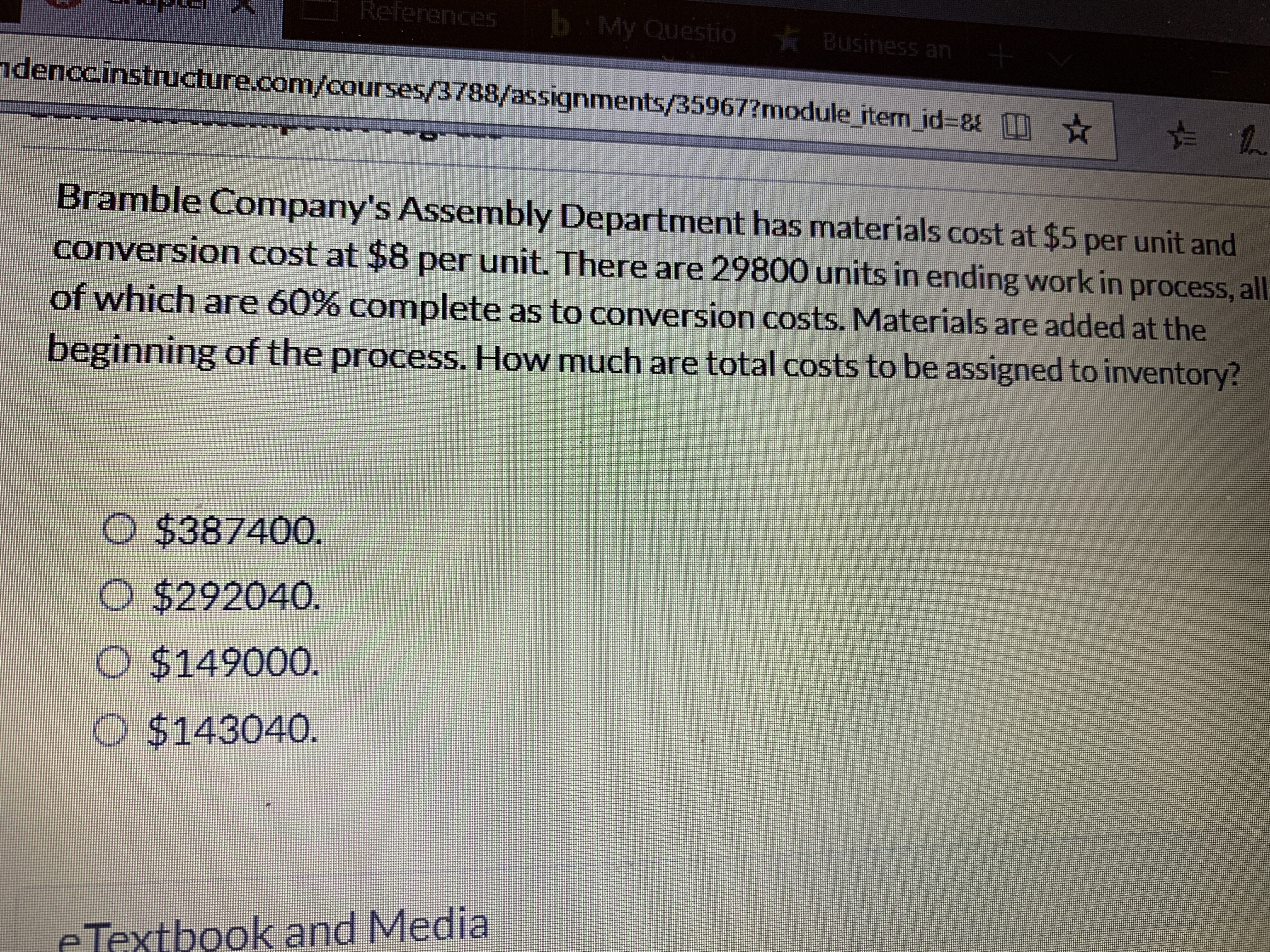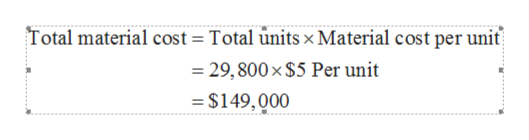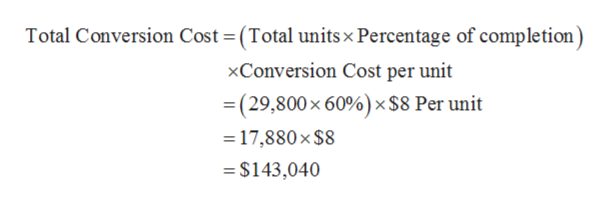# ReferencesMy QuestioBusiness anndenccinstructure.com/courses/3788/assignments/35967?module iten id-81Bramble Company's Assembly Department has materials cost at \$5 per unit andconversion cost at \$8 per unit. There are 29800 units in ending work in process, allof which are 60% complete as to conversion costs. Materials are added at thebeginning of the process. How much are total costs to be assigned to inventory?\$387400.O \$292040.\$149000.\$143040.e Textbook and Media

Question
9 viewshelp_outlineImage TranscriptioncloseReferences My Questio Business an ndenccinstructure.com/courses/3788/assignments/35967?module iten id-8 1 Bramble Company's Assembly Department has materials cost at \$5 per unit and conversion cost at \$8 per unit. There are 29800 units in ending work in process, all of which are 60% complete as to conversion costs. Materials are added at the beginning of the process. How much are total costs to be assigned to inventory? \$387400. O \$292040. \$149000. \$143040. e Textbook and Media fullscreen
check_circle

Step 1

Compute the amount of material cost by using the equation given above:

In the above equation, the amount of total material cost has been calculated by multiplying the total number of units with the material cost per unit as given in the case.help_outlineImage TranscriptioncloseTotal material cost = Total units x Material cost per unit = 29,800 x \$5 Per unit = \$149,000 fullscreen
Step 2

Compute the amount of conversion cost the equation given above:

In the above equation, the amount of conversion cost has been calculated by taking total unit...help_outlineImage TranscriptioncloseTotal Conversion Cost (Total units x Percentage of completion) xConversion Cost per unit =(29,800x 60%) x \$8 Per unit 17,880 x \$8 =\$143,040 fullscreen

### Want to see the full answer?

See Solution

#### Want to see this answer and more?

Solutions are written by subject experts who are available 24/7. Questions are typically answered within 1 hour.*

See Solution
*Response times may vary by subject and question.
Tagged in